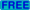# Course on Probabilistic Algorithms, Chiang-Mai, December 2004

By Philippe Flajolet. This is the outline of a set of 10 lectures for the Fifteenth Asian School on Computer Science, December 11-15, 2004 following the ASIAN'04 Conference. Chiang Mai, Thailand. This will be mostly a course on "selected topics" as no reasonably complete coverage of the vast topic of probabilistic algorithms can nowadays be expected. Several of the examples discussed will be related to the area of Massive Data Sets. The general orientation of the course will be to focus on analytic-combinatorial methods.
Here is a detailed plan and some relevant references. Most are freely available and markedbut this does not apply to two of the books.

## Probability, combinatorics, and algorithms

• What is a probability distribution? Distribution function, moments, probability generating function for discrete laws. The Bernoulli/binomial, geometric, and Poisson laws. Analogues for continuous laws. The exponential and Gaussian distribution. The inversion method for simulating a given distribution.
• What is a combinatorial model? Counting and generating functions. The ordinary and exponential generating functions. The multivariate versions. Examples: counting of trees and triangulations.
• Algorithms that bet on the likely shape of data. Analysis in the average-case and in probability. To be contrasted with randomized algorithms which purposely introduce randomness. The example of QuickSort and Randomized Quicksort. Binary search trees and their randomized version. Skip lists.
• Approximate counting: an example of a randomized algorithm whose analysis involves interesting combinatorial-analytic methods. More generally: counting regular languages and poles of rational functions.
Reading: Principally the books by Flajolet-Sedgewick and Motwani-Raghavan. Unfortunately they are only available commercially. The Vitter-Flajolet chapter is freely available on the web (though without figures, because of the technology of the time).
Standard texts on ALGORITHMS (only commercially available): these are only indirectly needed for the course, but anybody seriously interested in algorithms and data structures should have consulted them.

## Random allocations

• Elementary uses of exponential generating functions. Constrained functions from a finite set to a finite set (allocations).
• The birthday paradox and coupon collector problems. Hash tables that don't waste space or time are (nearly) impossible.
• The Poisson law for table occupancy. Use for paged hash tables: the expected worst-case of random alpha-full allocations.
• The "hit counting" algorithm of Kyu Young Whang et al.
• The log-log load balancing slick trick of Azar et al.
• Bloom filters.

## Digital trees and applications

• What is a digital tree aka "trie"? Basic data structure for searching. The probabilistic divide-and-conquer (here binomial/Bernoulli) recurrence. Exact solution by means of exponential generating functions.
• Elementary asymptotic methods for trie recurrences. Soft introduction to the Mellin technology. Application: Randomized leader election and the Tree Protocol.
• Straight sampling and adaptive sampling. Use of adaptive sampling as a cardinality estimator.

## Probabilistic counting and LogLog Counting

• Hash values and observables of multisets. The angel-daemon model.
• Basic ideas of Probabilistic Counting (by Flajolet-Martin).
• Basic ideas of LogLog Counting (by Durand-Flajolet).